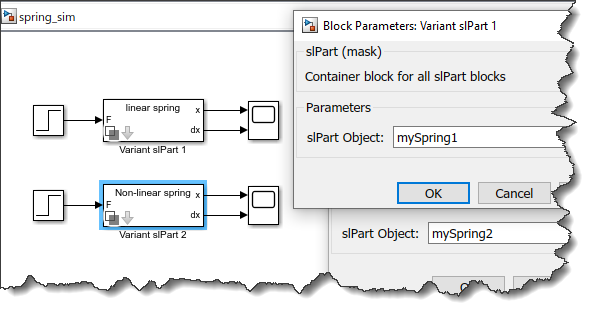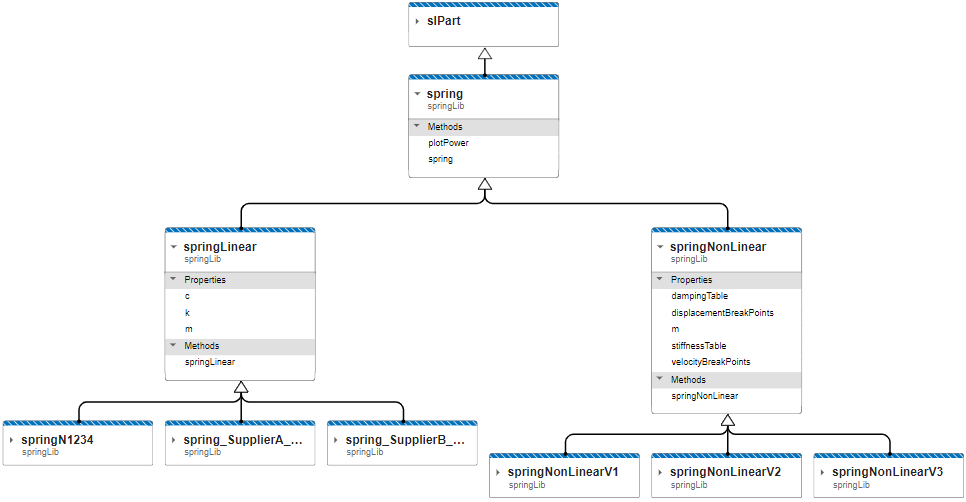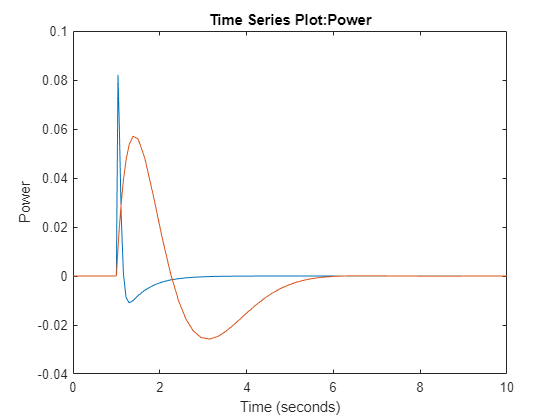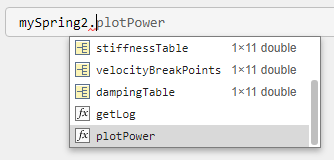# Unifying MATLAB and Simulink: A User Story Part 4

In today's post, I continue extending the framework introduced over the past few weeks. If you missed the previous posts in this series, here are links:
So far in this series, the framework I introduced has been mostly focused on configuring a model, with the mindset of simply "picking a part" by instantiating a MATLAB object from a library of MATLAB classes. In this post, I extend the idea of "Unifying MATLAB and Simulink" to managing and post-processing logged data after simulation.
By the end, I hope you will see how this slPart framework leads to fully contained components that include pre-defined parameterizations, variants, and functions/methods to interact with it in MATLAB. Assuming the slPart has been created and validated by a domain expert, it can then be shared with other users and simply used in a straightforward and easy to discover manner.

## A Method to Retrieve Logged Data

When I created the slPart class, I added a BlockPath property, mentioning that it was going to be useful later. It's now time to use it!
What I use BlockPath for is to retrieve the data logged inside the subsystem. For that, I create a method that takes a Simulink.SimulationOutput object and parses it to store the logged data in a newly added Log property of the slPart object. This method simply leverages the find method of the Simulink.SimulationData.Dataset object. Here is what the slPart class now looks like:
classdef slPart < handle
properties (Hidden = true)
BlockPath char
end
methods
% See previous posts
end

function obj = getLog(obj,out)
obj.Log = out.logsout.find('-regexp','BlockPath',[obj.BlockPath '(/.*)?\$']);
end

end
end
That way, if I simulate a model with multiple parts, each part will be able to retrieve its own data.
For example, let's put two copies of the spring with variants created in the previous post and parameterize them with different objects.Assign objects of different classes to each of them and simulate. Once the simulation is complete, each object can retrieve its own logged data:
mdl = 'spring_sim';
mySpring1 = springLib.springN1234;
mySpring2 = springLib.springNonLinearV1;
out = sim(mdl);
mySpring1.getLog(out);
mySpring2.getLog(out);
mySpring1.Log
ans =
Simulink.SimulationData.Dataset 'logsout' with 3 elements Name BlockPath ________ ________________________________________ 1 [1x1 Signal] position .../Variant slPart 1/linear spring/Integ 2 [1x1 Signal] velocity .../Variant slPart 1/linear spring/Integ 3 [1x1 Signal] force ...im/Variant slPart 1/linear spring/Sum - Use braces { } to access, modify, or add elements using index.
mySpring2.Log
ans =
Simulink.SimulationData.Dataset 'logsout' with 3 elements Name BlockPath ________ ________________________________________ 1 [1x1 Signal] position ...iant slPart 2/Non-linear spring/Integ 2 [1x1 Signal] velocity ...iant slPart 2/Non-linear spring/Integ 3 [1x1 Signal] force ...ariant slPart 2/Non-linear spring/Sum - Use braces { } to access, modify, or add elements using index.

## Defining Custom Post-Processing and Visualization

Now that each part is able to retrieve its own data, it becomes possible to define custom post-processing and visualization of the results for a part. To illustrate how this can work, I rearranged my example project a little bit to have both springLinear and springNonLinear subclassing the same spring superclass. Here is how the dependency of my classes now looks like in using the Class Diagram Viewer:
matlab.diagram.ClassViewer('Folders',currentProject().RootFolder);After this reorganization, I added a simple plotPower method to the spring class. Since each spring subsystem has two logged signals, named "velocity" and "force", I multiply them to create a "power" timeseries and plot it. Here is what the spring class looks like:
classdef spring < slPart
methods
function plotPower(obj)
power = obj.Log.get('velocity').Values*obj.Log.get('force').Values;
power.Name = 'Power';
plot(power)
end
end
end
Using the same model as above, I can then call this method for each object inheriting from the spring class.
mdl = 'spring_sim';
mySpring1 = springLib.springN1234;
mySpring2 = springLib.springNonLinearV1;
out = sim(mdl);
mySpring1.getLog(out);
mySpring2.getLog(out);
figure;
mySpring1.plotPower;
hold on
mySpring2.plotPower;